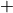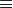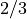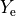# Parameter List by Number¶

Number

Name

Format

Unit

Synopsis

1

dt

float

sec

KEPLER timestep.

2

jm

integer

-

Number of zones.

3

dtold

float

sec

KEPLER timestep used for the previous cycle.

4

ncyc

integer

-

KEPLER cycle number.

5

fcr

float

-

Maximum fractional zonal radius change since the last iteration.

6

fct

float

-

Maximum fractional zonal temperature change since the last iteration.

7

iter

integer

-

Number of iterations taken for convergence.

8

told

float

sec

Time at the beginning of the last timestep.

9

tfcr

float

-

Total maximum fractional zonal radius change during current cycle.

10

tfct

float

-

Total maximum fractional zonal temperature change during current cycle.

11

fcl

float

-

Maximum fractional zonal luminosity change since the last iteration.

12

tfcl

float

-

Total maximum fractional zonal luminosity change since the last iteration.

13

idtcon

integer

-

Symbol of the ion most limiting the current KEPLER timestep.

14

imax

integer

-

Total number of ions in the APPROX network (usually 19).

15

nnet

integer

-

Number of ion networks specified.

16

numit

integer

-

Total number of ions specified in the generator (usually 19).

17

totm

float

g

Current total amount of mass in the problem (incl. summ0 (p 61)).

18

nreac

integer

-

Number of nuclear reactions edited (ions only – usually 29).

19

itert

integer

-

Total number of iterations since problem generation.

20

jfcr

integer

-

Zone with largest fractional radius change since the last iteration.

21

jfct

integer

-

Zone with largest fractional temperature change since the last iteration.

22

jfcl

integer

-

Zone with largest fractional luminosity change since the last iteration.

23

snuc

float

erg/g/sec

Current nuclear energy generation rate from last call to subroutine SDOT.

24

s0exp

float

erg/g

Neutrino deposition coefficient derived from the values of parameters jshell0 (p 93), jshell1 (p 94), and eexplode (p 95) and the current zonal of the star.

25

xlumn

float

erg/sec

Neutrino luminosity.

26

enini

float

erg

Total initial internal energy.

27

enink

float

erg

Total initial kinetic energy.

28

eninp

float

erg

Total initial potential energy.

29

enint

float

erg

Total initial energyenini (q 26)enink (q 27)eninp (q 28).

30

ensc

float

erg

Total energy deposited so far from input “source”. See xlum0 (p 62).

31

eni

float

erg

Total internal energy.

32

enk

float

erg

Total kinetic energy.

33

enp

float

erg

Total potential energy.

34

ent

float

erg

Total current energyeni (q 31)enk (q 32)enp (q 33).

35

epro

float

erg

Total net energy produced so far by nuclear reactions less neutrino losses.

36

enes

float

erg

Total non-neutrino energy that has so far escaped from the star’s surface.

37

enc

float

erg

Energy check.

38

enscd

float

erg/sec

Rate of energy deposition by input “source”. See xlum0 (p 62).

39

enid

float

erg/sec

Rate of change in the total internal energy.

40

enkd

float

erg/sec

Rate of change in the total kinetic energy.

41

enpd

float

erg/sec

Rate of change in the total potential energy.

42

entd

float

erg/sec

Rate of change in the total energy.

43

eprod

float

erg/sec

Total rate of nuclear energy production less neutrino losses.

44

enesd

float

erg/sec

Total rate of energy escape from the star (in photons).

45

encd

float

erg/sec

Total rate of change in the energy check.

46

xlum

float

erg/sec

Surface luminosity in electromagnetic radiation (enesd).

47

radius

float

cm

Photospheric radius corresponding to radius withoptical depth.

48

teff

float

K

Effective surface temperature at radius (q 47).

49

ncycb

integer

-

Current cycle number used by the BURN coprocessor (should be same as ncyc).

50

ncycbt

integer

-

Number of cycles since BURN co-processing was initiated (usually = ncyc).

51

nburnz

integer

-

Number of zones co-processed by BURN during current KEPLER cycle.

52

nburnzt

integer

-

Total number of zones co-processed by BURN since problem generation.

53

imaxb

integer

-

Number of isotopes in the currently specified BURN network (typically 149).

54

nnetb

integer

-

Number of specified BURN isotopic networks (usually 1).

55

numitb

integer

-

Total number of isotopes specified in the BURN generator (typically 149).

56

jlm

integer

-

Number of zones at the end of the last KEPLER cycle.

57

jbmax

integer

-

Maximum zone number for which BURN co-processing is now being done.

58

dtsub

float

-

Length of a BURN co-processing/BURN ion convection subcycle (see nsubcycm (p 264)).

59

jpist

integer

-

Index of the piston position now being used (see :ref:’link’ and cycle).

60

jpistm

integer

-

Total number of piston positions specified in the LINK file (see Link Input File).

61

velocty0

float

cm/sec

Velocity of inner problem boundary due to specified piston (see Link Input File).

62

tbounce

float

sec

Core bounce time. Set by the nflux card in the LINK file (see Link Input File).

63

fluxnu0

float

-

Neutrino flux parameter. Set by the nflux card in the LINK file (see Link Input File).

64

fluxnua

float

-

Neutrino flux parameter. Set by the nflux card in the LINK file (see Link Input File).

65

chi0

float

-

Neutrino flux parameter. Set by the nflux card in the LINK file (see Link Input File).

66

fluxnub

float

-

Neutrino flux parameter. Set by the nflux card in the LINK file (see Link Input File).

67

chil

float

-

Neutrino flux parameter. Set by the nflux card in the LINK file (see Link Input File).

68

fluxnuc

float

-

Neutrino flux parameter. Set by the nflux card in the LINK file (see Link Input File).

69

chi2

float

-

Neutrino flux parameter. Set by the nflux card in the LINK file (see Link Input File).

70

nye

integer

-

Index of the LINK-specified Ye now being utilized (see Link Input File and subroutine sdot).

71

nyem

integer

-

Total number of ye-card-specifiedvalues in the LINK file (see Link Input File).

72

idmptime

integer

-

Integer index of the next dump time (dump cycle number in subroutine DUMPQ).

73

nylib

integer

-

Number of distinct mass coordinates used so far in describing zoning (ylib entries).

74

nylib0

integer

-

Number of ylib entries (see nylib (q 73)) at the end of the last qq dump cycle.

75

ndatq

integer

-

Current number of zonal edit variables to be dumped(see subroutines DUMPQ & DUMP).

76

locqnext

integer

-

Location of the next open track in the current ‘qq’ dump file (see subroutine DUMPQ).

77

ndatl

integer

-

Current number of specified ‘look’ variables (see subroutine READQ and ADDLOOK).

78

eninr

float

erg

Initial rotational energy.

79

enr

float

erg

Current rotational energy.

80

anglint

float

erg*sec

Initial angular momentum.

81

anglt

float

erg*sec

Current total angular momentum.

82

xmlossr

float

g/sec

Current mass loss rate.

83

zfak

float

-

Computed metallicity multiplier on mass loss rate.

84

enn

float

erg

Total neutrino energy lost from the star.

85

enpist

float

erg

Total energy input by the piston.

86

enpistd

float

erg/sec

Energy input rate by the piston.

87

capeff

float

cm**2/g

88

xmlost

float

g

Mass lost in the “wind”.

89

radconv

float

cm

Radius outside of which no convection is allowed.

90

ninvl

integer

-

Number of BURN matrix inversions in last cycle.

91

ngbkup

integer

-

Number of negative abundance backups in subroutine SDOT for APPROX network.

92

mncbkup

integer

-

Number mass non-conservation backups in subroutine SDOT for APPROX network.

93

nacbkup

integer

-

Number of excess abundance change backups in subroutine SDOT for APPROX network.

94

isebkup

integer

-

Number if ISE non-convergence backups in subroutine SDOT.

95

enhd

float

-

Rate of change of thermal (“heat”) energy content.

96

pboundac

float

erg/cc

Boundary pressure due to accretion.

97

velnegm

float

-

This quantity is for fallback test only.

98

fbrate

float

-

Fallback accretion rate.

99

fallback

float

-

Total fallback mass.

100

ncycr

integer

-

Cycle of problem generation or last restart.

101

h1init

float

-

Initial central h1 mass fraction.

102

enrd

float

erg/sec

Rate of change of rotational energy during last step.

103

ilastpl

integer

-

”Time-of-day” seconds of last plot.

104

itimeg

integer

-

Time/date when problem was generated.

105

itimed

integer

-

Time/date when dump was generated.

106

entloss

float

-

Total energy removed from problems by cutsurf.

107

eniloss

float

-

Internal energy removed from problems by cutsurf.

108

enkloss

float

-

Kinetic energy removed from problems by cutsurf.

109

enploss

float

-

Potential energy removed from problems by cutsurf.

110

enrloss

float

-

Rotational energy removed from problems by cutsurf.

111

angloss

float

-

Total angular momentum removed from problems by cutsurf.

112

anglwnd

float

-

Total angular momentum lost due to wind.

113

angit

float

-

Total momentum of inertia of the star.

114

wimpcrsi

float

1/sec

WIMP spin-emph{indepenedent} capture rate.

115

wimpcrsd

float

1/sec

WIMP spin-emph{depenedent} capture rate.

116

wimparad

float

cm

117

wimpateq

float

sec

WIMP capture & annihilation equilibrium time scale.

118

wimparat

float

sec

WIMP annihilation rate (two WIMPs annihilate in one annihilation “event”).

119

wimpalum

float

erg/sec

Total WIMP annihilation luminosity as computed by WIMP subroutine in.

120

eprodw

float

erg/sec

WIMP energy deposition rate in star.

121

eprow

float

erg

Total energy deposited in star by WIMP annihilation.

122

eprodwx

float

erg/sec

WIMP annihilation luminosity emph{outside} star.

123

eprowx

float

erg

Total energy produced by wimps OUTSIDE star by WIMP annihilation.

124

xmacc

float

g

Total amount of mass accreted in.

125

dmacc

float

g

Mass accreted in last time step in.

126

jloss

integer

-

Mass losing zone.

127

jacc

integer

-

Accretion zone.

128

delmass

float

g

Mass lost in last time step in.

129

xmdec

float

g

Total mass removed from bottom.

130

decmass

float

g

Total mass removed from bottom and not added to substrate.

131

dmdec

float

g

Mass removed in last time step.

132

xmacrate

float

g/sec

Accretion rate.

133

he4init

float

-

Initial He4.

134

zinit

float

-

Initial metallicity.

135

xladv

float

erg/sec

136

dvloss

float

cc

Volume change due to mass loss.

137

dvacc

float

cc

Volume change due to mass accretion.

138

dvdev

float

cc

Volume change due to mass decretion (from bottom).

139

env

float

erg

Total viscous energy dissipated.

140

envd

float

erg/sec

Viscous energy dissipation rate in last time step.

141

envc

float

erg

Total viscous energy dissipation check.

142

envcd

float

erg/sec

Viscous energy dissipation rate check in last time step.

143

enpuls

float

erg

Energy depositied by pulsar.

144

enpulsd

float

erg/sec

Energy deposition rate by pulsar.

145

ennidec

float

erg

Energy depositied bydecay.

146

ennidecd

float

erg/sec

Energy deposition rate bydecay.

147

enxheatd

float

erg/sec

Total rate of energy deposition by extra heating.

148

enxheat

float

erg

Total energy deposition by extra heating.

149

jaccemx

integer

-

150

pboundat

float

erg/cc

Atmosphere surface boundary pressure resulting from use of isurf (p 523).

151

angltx

float

erg*sec

X-component of current total angular momentum.

152

anglty

float

erg*sec

Y-component of current total angular momentum.

153

angltz

float

erg*sec

Z-component of current total angular momentum.

154

anglossx

float

erg*sec

X-component of angular momentum removed from problems by cutsurf.

155

anglossy

float

erg*sec

Y-component of angular momentum removed from problems by cutsurf.

156

anglossz

float

erg*sec

Z-component of angular momentum removed from problems by cutsurf.

157

anglwndx

float

-

X-component of angular momentum lost due to wind.

158

anglwndy

float

-

Y-component of angular momentum lost due to wind.

159

anglwndz

float

-

Z-component of angular momentum lost due to wind.

160

iterbar

integer

-

Current iteration counter for problem termination.

161

xlumeff

float

erg/sec